• 先验信噪比单通道语音增强算法在信噪比较高时能有效地去除噪声，但在信噪比较低时语音高次谐波失真较为严重。针对此提出了一种基于谐波重构的先验信噪比估计算法，对增强后的信号加权求平方，进行功率谱的二次谱处理...
• ## 先验信噪比估计

千次阅读 2019-09-30 17:41:14
先验信噪比估计 很多降噪算法如维纳滤波、MMSE估计器等都依赖先验信噪比(priori SNR)信息 定义先验信噪比(priori SNR)、后验信噪比(posteriori SNR)如下 ξk(n)=E{Ak2(n)}λd(k,n)(1) \xi_{k}(n)=\frac{E\left\{A_{k...
先验信噪比估计
很多降噪算法如维纳滤波、MMSE估计器等都依赖先验信噪比(priori SNR)信息
定义先验信噪比(priori SNR)、后验信噪比(posteriori SNR)如下

ξ

k

(

n

)

=

E

{

A

k

2

(

n

)

}

λ

d

(

k

,

n

)

(1)

\xi_{k}(n)=\frac{E\left\{A_{k}^{2}(n)\right\}}{\lambda_{d}(k, n)}\tag1

γ

k

(

n

)

=

Y

k

2

(

n

)

λ

d

(

k

,

n

)

(2)

\gamma_k(n)=\frac{{Y_{k}^{2}(n)}}{\lambda_{d}(k, n)}\tag2

上式中的

A

k

(

n

)

A_{k}(n)

为目标信号的幅度谱、

λ

d

(

n

)

\lambda_d(n)

为噪声的功率谱，这里先假定噪声平稳且可以在无语音段估计得到。
假设噪声和语音不相关且为加性噪声，则

ξ

k

\xi_k

可以写成下式

ξ

k

(

n

)

=

E

{

A

k

2

(

n

)

}

λ

d

(

k

,

n

)

=

E

{

Y

k

2

(

n

)

−

λ

d

(

k

,

n

)

}

λ

d

(

k

,

n

)

=

E

{

Y

k

2

(

n

)

}

λ

d

(

k

,

n

)

−

1

=

E

{

λ

k

(

n

)

−

1

}

(3)

\begin{aligned}\xi_{k}(n)&=\frac{E\left\{A_{k}^{2}(n)\right\}}{\lambda_{d}(k, n)}\\&=\frac{E\left\{Y_{k}^{2}(n)-\lambda_d(k,n)\right\}}{\lambda_{d}(k, n)}\\&=\frac{E\left\{Y_{k}^{2}(n)\right\}}{\lambda_{d}(k, n)}-1\\&=E\left\{\lambda_{k}(n)-1\right\}\end{aligned}\tag3

一般所说的SNR就是指先验信噪比，维纳滤波器就是关于先验信噪比的函数

W

=

ξ

1

+

ξ

(4)

W=\frac{\xi}{1+\xi}\tag4

后验信噪比也叫瞬时信噪比，如谱减公式中用到的就是后验信噪比

A

2

=

∣

Y

∣

2

−

λ

d

=

1

λ

d

(

γ

−

1

)

(5)

\begin{aligned}A^2&=|Y|^2-\lambda_d\\&=\frac{1}{\lambda_d}(\gamma-1)\end{aligned}\tag5

因为谱减法是直接使用瞬时信噪比，因此会更容易产生音乐噪声，当然，也有很多的改进方法减小音乐噪声的影响。
合并(1)、(3)两式得到以下形式

ξ

k

(

n

)

=

E

{

1

2

A

k

2

(

n

)

λ

d

(

k

,

n

)

+

1

2

[

γ

k

(

n

)

−

1

]

}

(6)

\xi_k(n)=E\begin{Bmatrix}\frac{1}{2}\frac{A_{k}^{2}(n)}{\lambda_{d}(k, n)}+\frac{1}{2}[\gamma_k(n)-1]\end{Bmatrix}\tag6

这里还有一个烦人的期望符号

E

E

，还是老方法，用一阶递归平滑代替时间平均，则估计得到的

ξ

k

(

n

)

\xi_k(n)

为下式：

ξ

k

^

(

n

)

=

α

A

^

k

2

(

n

−

1

)

λ

d

(

k

,

n

−

1

)

+

(

1

−

α

)

m

a

x

(

γ

k

(

n

)

−

1

,

0

)

(7)

\hat{\xi_k}(n)=\alpha\frac{\hat{A}_k^2(n-1)}{\lambda_d(k,n-1)}+(1-\alpha)max(\gamma_k(n)-1,0)\tag7

其中

A

^

k

2

(

n

−

1

)

\hat{A}_k^2(n-1)

表示上一帧的幅度估计值。
(7)式就是著名的判决引导(Dicision-Directed)公式，被广泛用在了降噪算法的大街小巷里
例如，webrtc的单通道降噪算法源码里可以看到如下模块
// Compute prior and post SNR based on quantile noise estimation.
// Compute DD estimate of prior SNR.
// Inputs:
//   * |magn| is the signal magnitude spectrum estimate.
//   * |noise| is the magnitude noise spectrum estimate.
// Outputs:
//   * |snrLocPrior| is the computed prior SNR.
//   * |snrLocPost| is the computed post SNR.
static void ComputeSnr(const NoiseSuppressionC *self,
const float *magn,
const float *noise,
float *snrLocPrior, float *logSnrLocPrior,
float *snrLocPost) {
size_t i;

for (i = 0; i < self->magnLen; i++) {
// Previous post SNR.
// Previous estimate: based on previous frame with gain filter.
float previousEstimateStsa = self->magnPrevAnalyze[i] /
(self->noisePrev[i] + 0.0001f) * self->smooth[i];
// Post SNR.
snrLocPost[i] = 0.f;
if (magn[i] > noise[i]) {
snrLocPost[i] = magn[i] / (noise[i] + 0.0001f) - 1.f;
}
// DD estimate is sum of two terms: current estimate and previous estimate.
// Directed decision update of snrPrior.
snrLocPrior[i] = 2.f * (
DD_PR_SNR * previousEstimateStsa + (1.f - DD_PR_SNR) * snrLocPost[i]);
logSnrLocPrior[i] = logf(snrLocPrior[i] + 1.0f);
}  // End of loop over frequencies.
}

展开全文后验信噪比 webrtc
• 【语音去噪】基于先验信噪比的维纳滤波算法语音去噪matlab源码.md
• 2) 令先验信噪比 R k ( w ) = ∣ S k ( w ) ∣ 2 ∣ N k ( w ) ∣ 2 R_k(w)=\frac{|S_k(w)|^2}{|N_k(w)|^2} Rk​(w)=∣Nk​(w)∣2∣Sk​(w)∣2​，则（D-2）可以化简为： η k ( w ) = R k ( w ) 1 + R k ( w ) (D-3...
首先，k时刻w频点的幅值由语音信号和噪声信号组成，我们的目的是减少噪声那部分的幅值。假设k时刻w频点语音、噪声幅值比例为

η

k

(

w

)

∈

[

0

,

1

]

\eta_k(w)\in[0,1]

，干净语音信号为

S

k

(

w

)

S_k(w)

，噪声信号

N

k

(

w

)

N_k(w)

，那么，干净语音信号的功率谱为：

∑

w

∑

k

∣

S

k

(

w

)

∣

2

\sum_w \sum_k|S_k(w)|^2

，语音片段的噪声为

∣

S

k

(

w

)

∣

(

1

−

η

k

(

w

)

)

|S_k(w)|(1-\eta_k(w))

，非语音片段的噪声为

∣

N

k

(

w

)

∣

η

k

(

w

)

|N_k(w)|\eta_k(w)

，于是，噪声信号的功率谱为：

∑

w

∑

k

(

∣

N

k

(

w

)

∣

η

k

(

w

)

+

∣

S

k

(

w

)

∣

(

1

−

η

k

(

w

)

)

)

2

\sum_w \sum_k(|N_k(w)|\eta_k(w)+|S_k(w)|(1-\eta_k(w)))^2

，假设噪声信号和语音信号不相关，噪声信号的功率谱可以化简为：

∑

w

∑

k

∣

N

k

(

w

)

∣

2

η

k

2

(

w

)

+

∣

S

k

(

w

)

∣

2

(

1

−

η

k

(

w

)

)

2

\sum_w \sum_k|N_k(w)|^2\eta_k^2(w)+|S_k(w)|^2(1-\eta_k(w))^2

​ 因此，整体的信噪比R可以定义为：

R

=

∑

w

∑

k

∣

S

k

(

w

)

∣

2

∑

w

∑

k

∣

N

k

(

w

)

∣

2

η

k

2

(

w

)

+

∣

S

k

(

w

)

∣

2

(

1

−

η

k

(

w

)

)

2

(D-1)

R=\frac{\sum_w \sum_k|S_k(w)|^2}{\sum_w \sum_k|N_k(w)|^2\eta_k^2(w)+|S_k(w)|^2(1-\eta_k(w))^2}\tag{D-1}

所以现在目标很明确，就是要让整体的信噪比R最大。此时将（D-1）对

η

k

(

w

)

\eta_k(w)

求导并等于0，求得信噪比最大时的

η

k

(

w

)

\eta_k(w)

值：
由求导公式：

(

u

v

)

′

=

u

′

v

−

u

v

′

v

2

(\frac{u}{v})^{'}=\frac{u^{'}v-uv^{'}}{v^2}

得：

R

′

=

−

(

∑

w

∑

k

∣

S

k

(

w

)

∣

2

)

(

2

∣

N

k

(

w

)

∣

2

η

k

(

w

)

−

2

∣

S

k

(

w

)

∣

2

(

1

−

η

k

(

w

)

)

)

(

∑

w

∑

k

∣

N

k

(

w

)

∣

2

η

k

2

(

w

)

+

∣

S

k

(

w

)

∣

2

(

1

−

η

k

(

w

)

)

2

)

2

=

0

⇓

−

(

∑

w

∑

k

∣

S

k

(

w

)

∣

2

)

(

2

∣

N

k

(

w

)

∣

2

η

k

(

w

)

−

2

∣

S

k

(

w

)

∣

2

(

1

−

η

k

(

w

)

)

)

=

0

⇓

∣

N

k

(

w

)

∣

2

η

k

(

w

)

=

∣

S

k

(

w

)

∣

2

(

1

−

η

k

(

w

)

)

⇓

η

k

(

w

)

=

∣

S

k

(

w

)

∣

2

∣

N

k

(

s

)

∣

2

+

∣

S

k

(

w

)

∣

2

(D-2)

\begin{aligned}R^{'}=\frac{-(\sum_w \sum_k|S_k(w)|^2)(2|N_k(w)|^2\eta_k(w)-2|S_k(w)|^2(1-\eta_k(w)))}{(\sum_w \sum_k|N_k(w)|^2\eta_k^2(w)+|S_k(w)|^2(1-\eta_k(w))^2)^2}&=0\\ &\Downarrow\\ -(\sum_w \sum_k|S_k(w)|^2)(2|N_k(w)|^2\eta_k(w)-2|S_k(w)|^2(1-\eta_k(w)))&=0\\ &\Downarrow\\ |N_k(w)|^2\eta_k(w)&=|S_k(w)|^2(1-\eta_k(w))\\ &\Downarrow\\ \eta_k(w)&=\frac{|S_k(w)|^2}{|N_k(s)|^2+|S_k(w)|^2} \end{aligned}\tag{D-2}

令先验信噪比

R

k

(

w

)

=

∣

S

k

(

w

)

∣

2

∣

N

k

(

w

)

∣

2

R_k(w)=\frac{|S_k(w)|^2}{|N_k(w)|^2}

，则（D-2）可以化简为：

η

k

(

w

)

=

R

k

(

w

)

1

+

R

k

(

w

)

(D-3)

\eta_k(w)=\frac{R_k(w)}{1+R_k(w)}\tag{D-3}

从（D-3）可以定性分析出，维纳滤波器会对频谱上信噪比高的部分保持较大的权重，同时对信噪比低的部分进行抑制。如下图所示展开全文• 一种基于改进非因果先验信噪比的语音增强算法，戴荣涛，严勤，本文提出一种改进的非因果先验信噪比估计的最小对数均方误差的短时谱估计算法。算法用倒谱平滑来改进先验信噪比估计，由于非因果语音增强
• 本章提出了一种语音增强算法，该算法以基于先验信噪比估计的维纳滤波法为基础。通过计算无声段的统计平均得到初始噪声功率谱,并平滑处理初始噪声功率谱和带噪语音功率谱，更新了噪声功率谱；最后，考虑了某频率点处...
一、简介
本章提出了一种语音增强算法，该算法以基于先验信噪比估计的维纳滤波法为基础。通过计算无声段的统计平均得到初始噪声功率谱,并平滑处理初始噪声功率谱和带噪语音功率谱，更新了噪声功率谱；最后，考虑了某频率点处噪声急剧增大的情况，做了相关验证，该算法能有效地抑制变化范围不大或是稳定的噪声，但是对实际中的变化范围很广的噪声效果不是很好。 1、语音增强概述 1.1 语音增强的相关概念 嵌在语音系统中，语音信号不可避免的会受到周围噪声的干扰，从而影响语音的质量与可懂度。 语音增强：其实就是带噪语音中提取尽可能纯净的语音，改善语音质量和可懂度，提高噪声环境下语音通信系统的性能。 噪声都随机产生的，不可能完全消除。语音增强的目标是：减弱噪声、消除背景噪声、改进语音质量、使听着乐于接受，提高语音可懂度。 1.2 语音增强的相关算法 由于噪声来源众多，特性各不相同。语音增强处理系统的应用场合千差万别。 因此，不存在一种可以通用于各种噪声环境的语音增强算法。针对不同的环境，采取不同的语音增强算法。 语音增强算法按处理方式可以分为：基于语音周期性的增强算法，基于全极点模型的增强算法，基于短时谱估计的增强算法，基于信号子空间的增强算法和 基于HMM的增强算法。 从目前的发展来看，基于短时谱估计的方法是最有效的方法。具体包括谱减法、维纳滤波、最小均方误差短时谱幅度估计法（MMSE-STSA）和最小均方误差对数谱幅度估计法（MMSE-LSA）。本文主要讨论使用维纳滤波器实现语音的增强处理。 2 基于先验信噪比估计的维纳滤波语音增强理论 先验信噪比是语音增强算法中非常重要的参数。 通过Ephraim和 Malah提出的“直接判决”估计来计算先验信噪比的方法是最有效的和最容易计算的。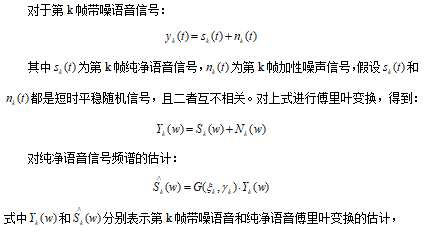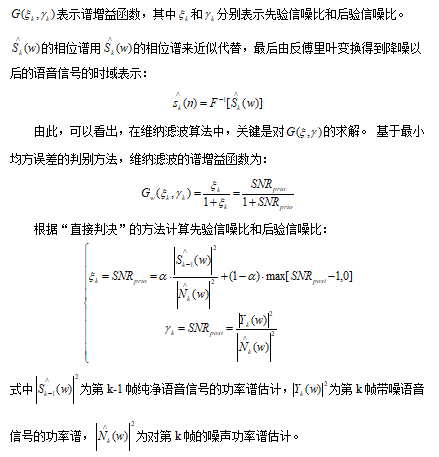二、源代码
clear all; clc; close all;

[xx, fs] = wavread('C5_3_y.wav');           % 读入数据文件
xx=xx-mean(xx);                         % 消除直流分量
x=xx/max(abs(xx));                      % 幅值归一化
IS=0.25;                                % 设置前导无话段长度
wlen=200;                               % 设置帧长为25ms
inc=80;                                 % 设置帧移为10ms
SNR=5;                                  % 设置信噪比SNR
NIS=fix((IS*fs-wlen)/inc +1);           % 求前导无话段帧数
alpha=0.95;

signal=awgn(x,SNR,'measured','db');               % 叠加噪声
output=Weina_Im(x,wlen,inc,NIS,alpha) ;
output=output/max(abs(output));
len=min(length(output),length(x));
x=x(1:len);
signal=signal(1:len);
output=output(1:len);

snr1=SNR_Calc(x,signal);            % 计算初始信噪比
snr2=SNR_Calc(x,output);            % 计算降噪后的信噪比
snr=snr2-snr1;
fprintf('snr1=%5.4f   snr2=%5.4f   snr=%5.4f\n',snr1,snr2,snr);

% 作图
time=(0:len-1)/fs;                        % 设置时间
subplot 311; plot(time,x,'k'); grid; axis tight;
title('纯语音波形'); ylabel('幅值')
subplot 312; plot(time,signal,'k'); grid; axis tight;
title(['带噪语音 信噪比=' num2str(SNR) 'dB']); ylabel('幅值')
function frameout=enframe(x,win,inc)

nx=length(x(:));            % 取数据长度
nwin=length(win);           % 取窗长
if (nwin == 1)              % 判断窗长是否为1，若为1，即表示没有设窗函数
len = win;               % 是，帧长=win
else
len = nwin;              % 否，帧长=窗长
end
if (nargin < 3)             % 如果只有两个参数，设帧inc=帧长
inc = len;
end
nf = fix((nx-len+inc)/inc); % 计算帧数
frameout=zeros(nf,len);            % 初始化
indf= inc*(0:(nf-1)).';     % 设置每帧在x中的位移量位置
inds = (1:len);             % 每帧数据对应1:len
frameout(:) = x(indf(:,ones(1,len))+inds(ones(nf,1),:));   % 对数据分帧
if (nwin > 1)               % 若参数中包括窗函数，把每帧乘以窗函数
w = win(:)';            % 把win转成行数据
function frameout=filpframe(x,win,inc)

[nf,len]=size(x);
nx=(nf-1) *inc+len;                 %原信号长度
frameout=zeros(nx,1);
nwin=length(win);                   % 取窗长
if (nwin ~= 1)                           % 判断窗长是否为1，若为1，即表示没有设窗函数
winx=repmat(win',nf,1);
x=x./winx;                          % 除去加窗的影响
x(find(isinf(x)))=0;                %去除除0得到的Inf
end

for i=1:nf
start=(i-1)*inc+1;
xn=x(i,:)';
sig(start:start+len-1)=sig(start:start+len-1)+xn;
end
三、运行结果展开全文matlab 算法 语音识别
•  噪声抑制算法中，谱减算法用的是后验证信噪比，维纳滤波器使用的是先验信噪比，MMSE（最小均方误差）算法既用到了先验信噪比，也用到了后验信噪比，那么，自然提出一个问题，在降噪过程中，先验信噪比与后 ...


噪声抑制算法中，谱减算法用的是后验证信噪比，维纳滤波器使用的是先验信噪比，MMSE（最小均方误差）算法既用到了先验信噪比，也用到了后验信噪比，那么，自然提出一个问题，在降噪过程中，先验信噪比与后验信噪比到底那个作用比较大。这个结论其实通过验证可以得出，先验信噪比是影响噪声抑制的主要参数，后验信噪比是辅助参数。

那么先验信噪比与后验信噪比它们之间又有什么关系，这里做一个分析。
先看下面的一个公式：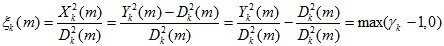这里k为帧数，m为频率，先验信噪比等于纯净语音信号的功率（X）除以噪声信号的功率（D），假设语音信号是平稳的，且噪声与语音信号不相关，则等于带噪语音功率（Y）减去噪声功率（D）后除以噪声功率，最后化简为先验信噪比等于后验信噪（gamma）比减去1。

另外，根据统计学中的先验信噪比与后验信噪比的关系，已知：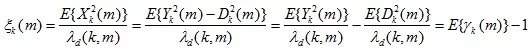这里，我们发现，第二个公式跟第一个是很像的，如果我们把这两个公式中的值分别只取一半进行中和一下，可以得到第三个公式：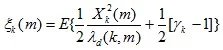再扩展下，如果这里的权重1/2变成一个变量a，上式就变为：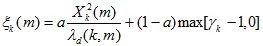这个公式就是知名的判决引导法公式，很多降噪算法就使用这个公式进行先验信噪比的估计！

参考文献：https://user.qzone.qq.com/314138065/blog/1442843834

致谢: 本文转载于 https://blog.csdn.net/fangbingxiao/article/details/80037628
展开全文语音增强 概率计算
• 算法采用非因果先验信噪比估计法来估计先验信噪比，并引入无语音概率的思想，对增益函数进行改进。实验结 果表明，相比传统MMSE-LSA算法，改进算法能更好地抑制残留噪声，提高语音的信噪比，增强效果更好。
• 本章提出了一种语音增强算法，该算法以基于先验信噪比估计的维纳滤波法为基础。通过计算无声段的统计平均得到初始噪声功率谱,并平滑处理初始噪声功率谱和带噪语音功率谱，更新了噪声功率谱；最后，考虑了某频率点处...matlab 算法 语音识别
• 结合经典的谱增益算法和迭代算法,提出了一种基于谱增益迭代的先验信噪比估计语音增强算 法。也就是通过谱增益的循环迭代,实现纯净语音信号估计逐步逼近真正的纯净语音,然后根据先 验信噪比定义更新先验信噪比的估计...
• 描述了先验信噪比估计的维纳滤波算法,分析了小波多分辨率分析在信号频谱划分中的作用,提出一种小波和先验信噪比维纳滤波相结合的改进算法。通过小波变换对带噪语音信号进行多尺度分解,然后对不同尺度的小波系数采用...
• 在DCT域提出了一种改进STNR (M-STNR)算法，其在原算法的第二步中利用信号的高斯模型假设条件，采用MMSE估计来获取当前帧先验信噪比的估计，算法无需语音增强系统增益因子的任何先验条件，在保持简单结构的同时避免...
• 噪声抑制算法中，谱减算法用的是后验证信噪比，维纳滤波器使用的是先验信噪比，MMSE算法既用到了先验信噪比，也用到了后验信噪比，那么，自然提出一个问题，在降噪过程中，先验信噪比与后验信噪比到底那个作用比较大...后验信噪比 噪声抑制
• 电信设备-基于Kalman的先验信噪比估计方法、装置及噪声抑制方法.zip
• 在基于先验信噪比的维纳滤波语音增强算法的基础上，结合语音端点检测算法，本文提出一种新算法。新算法在语音端点检测的基础上，通过平滑处理更新噪声信号功率谱以适应噪声不稳定的环境；通过计算有声段噪声信号估计...
• 如需转载，请注明出处！...核心思想：利用噪声平滑估计，结合MMSE-STSA的 Decision-Directed推导出的先验信噪比估计方法，应用进Wiener滤波去噪或谱减法去噪算法中。 给出结论：
• 当α→1时ξm,k会近似于前一帧的先验信噪比估计值，所以α的作用在于将相邻两帧估计的先验信噪比进行平滑加权。 从上面公式得知，DD算法的估计结果是ξm,kDD估计出的是第m-1帧的语音频谱分量的先验信噪比，而实际...
• ## SRN——信噪比

千次阅读 2018-03-27 19:28:58
这里不妨先用 matlab 所支持的函数对象（函数式编程）定义这样一个函数变量，可作为函数的参数进行传递，使用等：SNR = @(signal, noisy) 20*log10(norm(signal)/norm(signal-noisy)) ...
•研究论文
• 能量检测由于不需要授权用户的先验信息而被广泛应用。然而由于接收的噪声存在不确定性，使得在信噪比低于某一闸值时，无论观测时间多长，都无法保证检测结果满足要求的检测性能，这一闸值被称作"信噪比墙"。本文通过...
• 针对语音增强技术中先验信噪比参数的估计问题,本文通过结合两步噪声消除技术以及语音与噪声分量的高斯统计模型,在频率域中提出了一种新的先验信噪比估计算法。该算法基于直接判决方法的输出结果,利用最小均方误差...
• ## 浅谈VMD(变分模态分解)

万次阅读 多人点赞 2019-12-02 11:41:40
对于预定义模态数K的解决方法，推荐一篇论文：基于反馈变分模式分解的单通道盲源分离算法，他采用反馈模式对K自动识别定义，解除了预定义的先验模式。 EMD以及VMD的matlab代码（求大家给个赞别） VMD链接: ...变分模态分解VMD 信号处理
• PICHCS利用多光谱图像的空间相关性和谱间相关性重建出初始图像，并将相邻谱段的初始图像取平均获得高信噪比先验图像。先验图像与目标图像相减可以使优化目标稀疏化，并使得重建结果具有与先验图像类似的高信噪比...
• 基于不同信噪比的模拟数据，对提出方法、基于种子点的方法、独立成分分析方法以及两种聚类方法（归一化最小化割和K-均值方法）进行比较。基于真实脑静息态功能核磁共振数据，使用提出方法对默认模式网络进行提取。...磁共振成像 脑功能网络 半监督聚类...